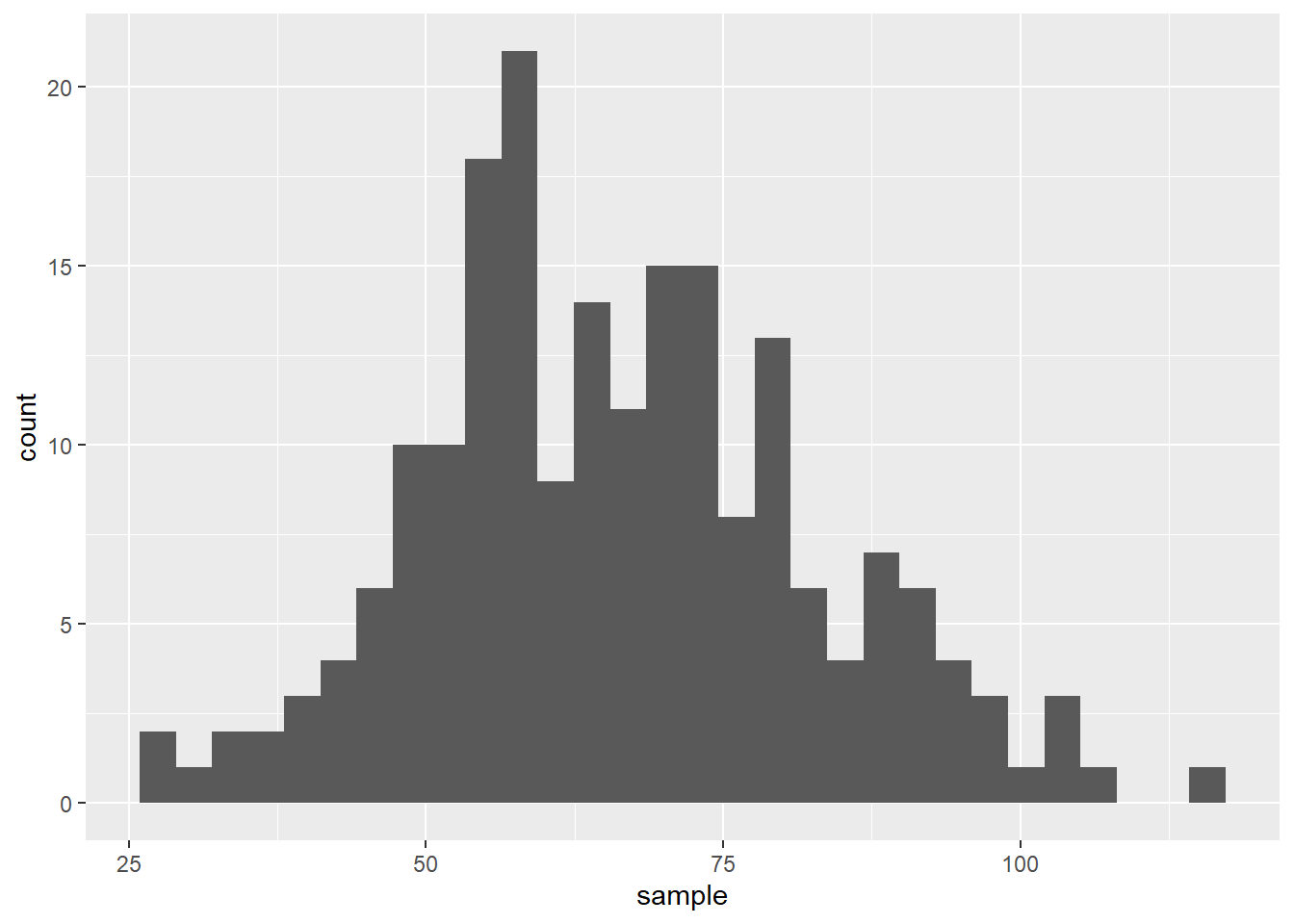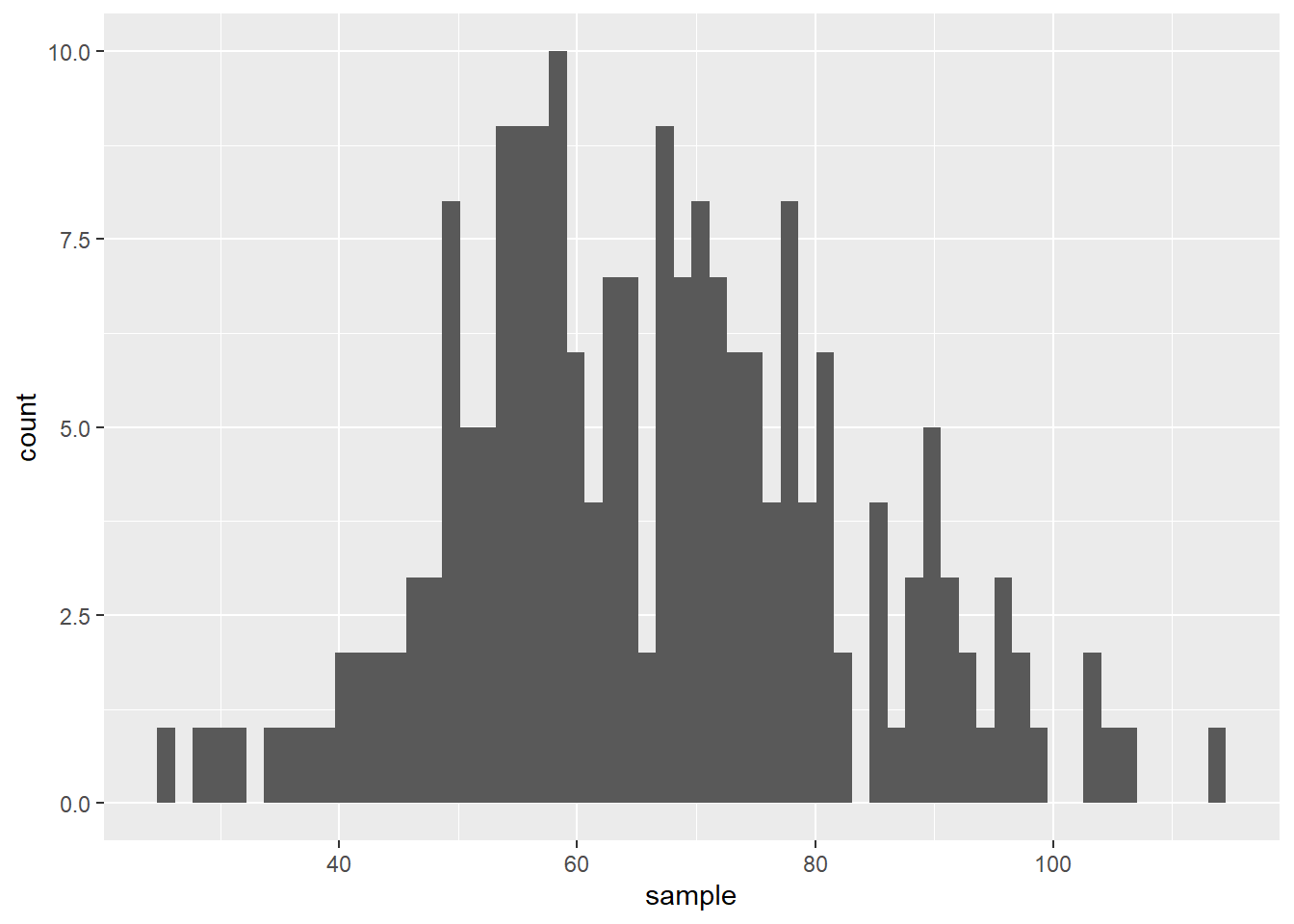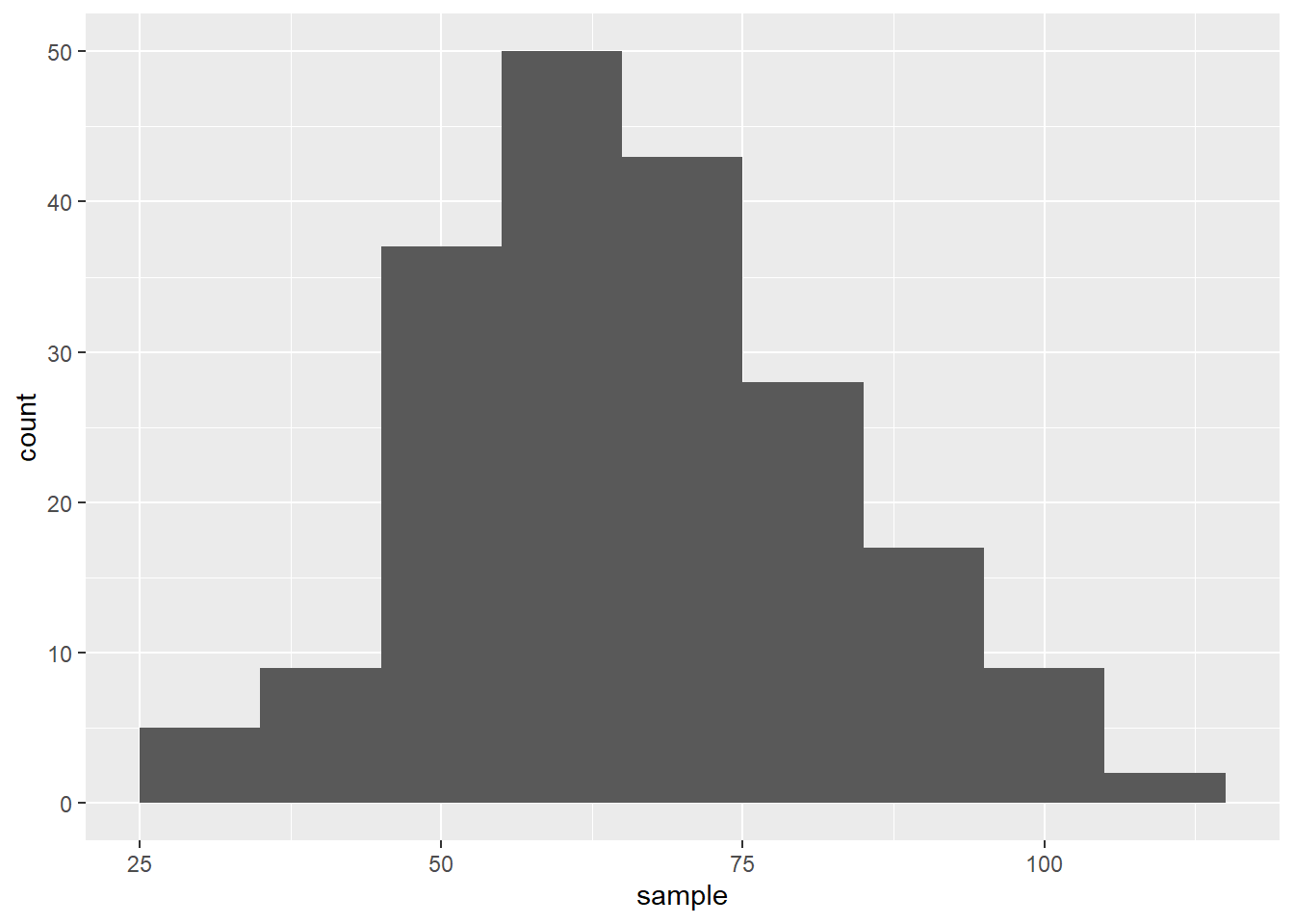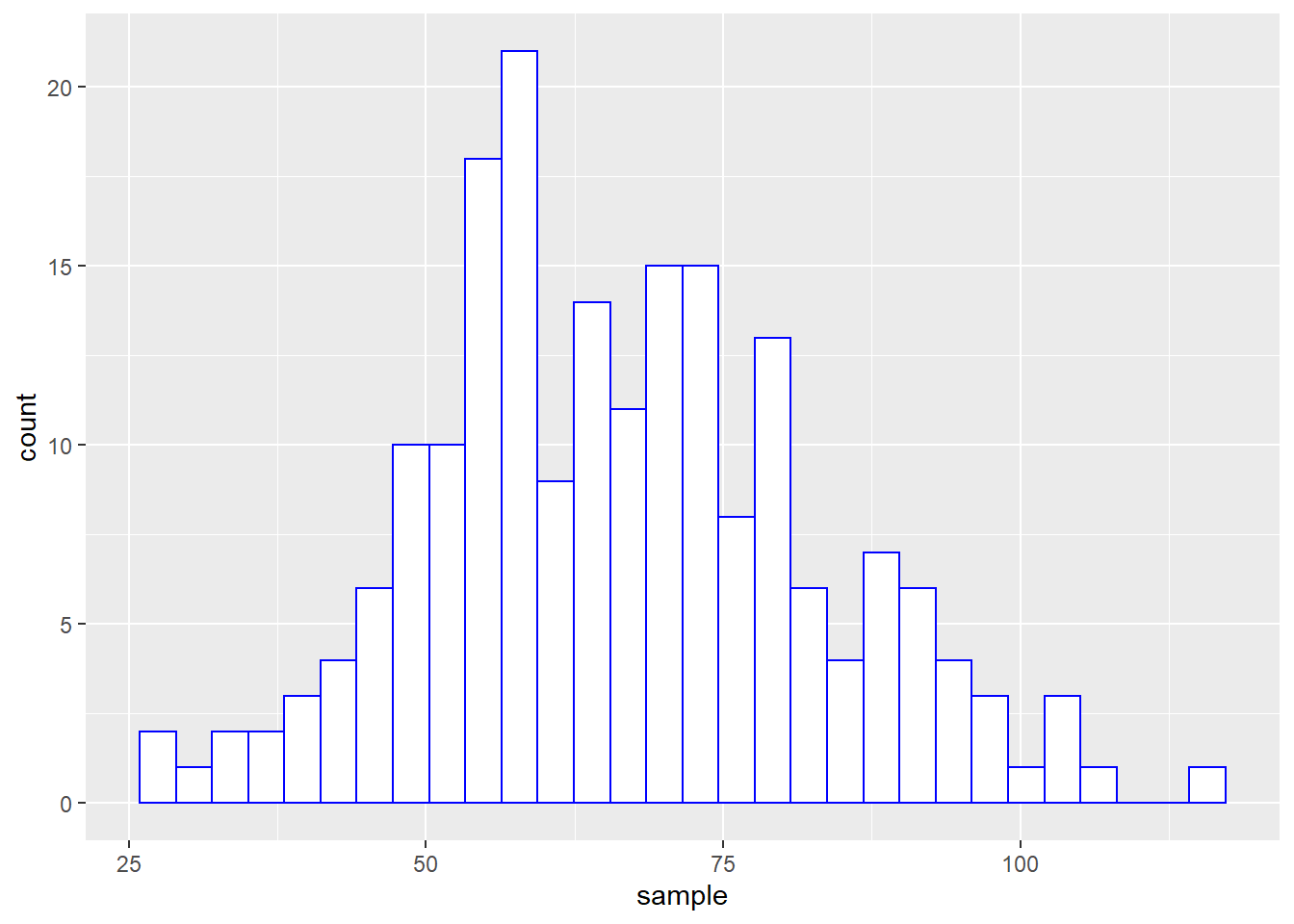A histogram of frequency represents the distribution of a sample. It looks like a bar chart which X-axis shows the values of the data series and which Y-axis counts the occurrences of these values. Here we see how to use ggplot() to draw a simple histogram using a random, normally distributed dataset. The random, normally-distributed values will be generated by rnorm()(see here for more info about this function).

Let’s take a simple example to see how to build a scatter plot with ggplot().

# dataframe
df <- data.frame(ID,sample)
str(df)
## 'data.frame':    200 obs. of  2 variables:
##  $ID : int 1 2 3 4 5 6 7 8 9 10 ... ##$ sample: num  34.4 63.5 59.3 47.1 63.7 ...

Now, let’s map the data from the sample by typing ggplot(df, aes(sample)) and use geom_histogram() to draw the bars:

ggplot(df, aes(sample)) +
geom_histogram()
## stat_bin() using bins = 30. Pick better value with binwidth.Note that the console displays a warning message. This warning tells you that ggplot2 has split your plot in 30 bars (or bins) by default. It is up to you to define whether or not this was a good way to represent your data. You are strongly advised to try several options, with fewer or more bins. To define a specific bin number, use bins= inside geom_histogram(); here is an example with 60 bins:

ggplot(df, aes(sample)) +
geom_histogram(bins = 60)You may as well indicate a specific binwidth using binwidth=. Here is an example with a binwidth of 10:

ggplot(df, aes(sample)) +
geom_histogram(binwidth = 10)A histogram may be visually enhanced with a bit of color and frames. For instance, you may change the color of the bars, and/or highlight them with a colored frame. Use color= and fill= to achieve the result that you want:

ggplot(df, aes(sample)) +
geom_histogram(color="blue", fill="white")
## stat_bin() using bins = 30. Pick better value with binwidth.### Adding plot title, axis titles, ticks, labels and other essential elements

In this section, you will learn how to set/modify all the necessary elements that make a plot complete and comprehensible. Such elements are: